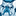# QlikView App Dev

Discussion Board for collaboration related to QlikView App Development.

Announcements
May 18th 10AM ET, Live Chat, bring your QlikView questions. REGISTER
cancel
Showing results for
Did you mean:Contributor III

## Row totals

I'm quiet new on this and maby have a simple question:  In a Pivot table I have a row with a condition: If(Column(5)=0, Column(1),0)  But the row totals applies the same condition instead of summing the rows indivdual results.  I have been searching and found several solutions about If and Aggr, but have not been able to get teh row's total correctly calculated.  Please advise if anybody has a solution!  Best regards

33 RepliesChampion III

May be try this?

= Sum(Aggr(IF(If(Column(5)=0, Column(1),0) , yourdimensionshere))Contributor III
Author

I get an error in the expressionn. Some parentheiss missing? I've tried to correct it, but without succes...Champion IIIContributor III
Author

= Sum(Aggr(IF(If(Column(5)=0, Column(1),0) , Origen))Champion III

Can you replace those Column(5) and Column(1) with your actual expressions and see if that works.Contributor III
Author

If(Column(5)=0, Column(1),0)

is

If(Sum ({<Y= {\$(=Max(Y)-1)}>}[Venta Neta])=0,Sum ({<Y= {\$(=Max(Y))}>}[Venta Neta]),0)

So, I should try

= Sum(Aggr(IF(If(Sum ({<Y= {\$(=Max(Y)-1)}>}[Venta Neta])=0,Sum ({<Y= {\$(=Max(Y))}>}[Venta Neta]),0) , Origen))

But I still get an error in the expression. Isn't a ) missing??Contributor III
Author

I've tried:

= Sum(Aggr(IF(If(Sum ({<Y= {\$(=Max(Y)-1)}>}[Venta Neta])=0,Sum ({<Y= {\$(=Max(Y))}>}[Venta Neta]),0) , Origen),0))

in order to add to the first If an alternative value

Expression is no okl but the result isn't!Master II

I just modified Nagaraju expression.. Can you see if the below works ?

= Sum(Aggr(IF(If(Column(5)=0, Column(1) ),0) , yourdimensionshere))

OR

= Sum(Total IF(If(Column(5)=0, Column(1) ),0) )Champion III

Try this?

= Sum(Aggr(If(Sum ({< Y= {\$(= Max(Y)-1)} >}[Venta Neta]) = 0,Sum ({< Y = {\$(=Max(Y))} >}[Venta Neta]),0), Origen))